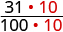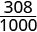# 1.8 The real numbers  (Page 6/13)

 Page 6 / 13
 0.31 0.308 Convert to fractions. $\frac{31}{100}$ $\frac{308}{1000}$ We need a common denominator to compare them.$\frac{310}{1000}$ $\frac{308}{1000}$

Because 310>308, we know that $\frac{310}{1000}>\frac{308}{1000}.$ Therefore, 0.31>0.308.

Notice what we did in converting 0.31 to a fraction—we started with the fraction $\frac{31}{100}$ and ended with the equivalent fraction $\frac{310}{1000}.$ Converting $\frac{310}{1000}$ back to a decimal gives 0.310. So 0.31 is equivalent to 0.310. Writing zeros at the end of a decimal does not change its value!

$\frac{31}{100}=\frac{310}{1000}\phantom{\rule{1em}{0ex}}\text{and}\phantom{\rule{1em}{0ex}}0.31=0.310$

We say 0.31 and 0.310 are equivalent decimals    .

## Equivalent decimals

Two decimals are equivalent if they convert to equivalent fractions.

We use equivalent decimals when we order decimals.

The steps we take to order decimals are summarized here.

## Order decimals.

1. Write the numbers one under the other, lining up the decimal points.
2. Check to see if both numbers have the same number of digits. If not, write zeros at the end of the one with fewer digits to make them match.
3. Compare the numbers as if they were whole numbers.
4. Order the numbers using the appropriate inequality sign.

Order $0.64___0.6$ using $<$ or $>.$

## Solution

$\begin{array}{cccccc}\begin{array}{c}\text{Write the numbers one under the other,}\hfill \\ \text{lining up the decimal points}.\hfill \end{array}\hfill & & & & & \begin{array}{c}\phantom{\rule{1.4em}{0ex}}0.64\hfill \\ \phantom{\rule{1.4em}{0ex}}0.6\hfill \end{array}\hfill \\ \\ \\ \begin{array}{c}\text{Add a zero to 0.6 to make it a decimal}\hfill \\ \text{with 2 decimal places.}\hfill \end{array}\hfill & & & & & \hfill \begin{array}{c}\hfill 0.64\hfill \\ \hfill 0.60\hfill \end{array}\hfill \\ \text{Now they are both hundredths.}\hfill & & & & & \\ \\ \\ \text{64 is greater than 60.}\hfill & & & & & \hfill 64>60\hfill \\ \\ \\ \text{64 hundredths is greater than 60 hundredths.}\hfill & & & & & \hfill 0.64>0.60\hfill \\ \\ \\ & & & & & \hfill 0.64>0.6\hfill \end{array}$

Order each of the following pairs of numbers, using $<\phantom{\rule{0.2em}{0ex}}\text{or}\phantom{\rule{0.2em}{0ex}}>\text{:}\phantom{\rule{0.2em}{0ex}}0.42___0.4.$

>

Order each of the following pairs of numbers, using $<\phantom{\rule{0.2em}{0ex}}\text{or}\phantom{\rule{0.2em}{0ex}}>\text{:}\phantom{\rule{0.2em}{0ex}}0.18___0.1.$

>

Order $0.83___0.803$ using $<$ or $>.$

## Solution

$\begin{array}{cccccc}& & & & & 0.83___0.803\hfill \\ \\ \\ \begin{array}{c}\text{Write the numbers one under the other,}\hfill \\ \text{lining up the decimals.}\hfill \end{array}\hfill & & & & & \begin{array}{c}0.83\hfill \\ 0.803\hfill \end{array}\hfill \\ \\ \\ \begin{array}{c}\text{They do not have the same number of}\hfill \\ \text{digits.}\hfill \end{array}\hfill & & & & & \begin{array}{c}0.830\hfill \\ 0.803\hfill \end{array}\hfill \\ \text{Write one zero at the end of}\phantom{\rule{0.2em}{0ex}}0.83.\hfill & & & & & \\ \\ \\ \begin{array}{c}\text{Since}\phantom{\rule{0.2em}{0ex}}830>803,830\phantom{\rule{0.2em}{0ex}}\text{thousandths is}\hfill \\ \text{greater than 803 thousandths.}\hfill \end{array}\hfill & & & & & 0.830>0.803\hfill \\ \\ \\ & & & & & 0.83>0.803\hfill \end{array}$

Order the following pair of numbers, using $<\phantom{\rule{0.2em}{0ex}}\text{or}\phantom{\rule{0.2em}{0ex}}>\text{:}\phantom{\rule{0.2em}{0ex}}0.76___0.706.$

>

Order the following pair of numbers, using $<\phantom{\rule{0.2em}{0ex}}\text{or}\phantom{\rule{0.2em}{0ex}}>\text{:}\phantom{\rule{0.2em}{0ex}}0.305___0.35.$

<

When we order negative decimals, it is important to remember how to order negative integers. Recall that larger numbers are to the right on the number line. For example, because $-2$ lies to the right of $-3$ on the number line, we know that $-2>-3.$ Similarly, smaller numbers lie to the left on the number line. For example, because $-9$ lies to the left of $-6$ on the number line, we know that $-9<-6.$ See [link] .

If we zoomed in on the interval between 0 and $-1,$ as shown in [link] , we would see in the same way that $-0.2>-0.3\phantom{\rule{0.2em}{0ex}}\text{and}\phantom{\rule{0.2em}{0ex}}-0.9<-0.6.$

Use $<$ or $>$ to order $-0.1___-0.8.$

## Solution

$\begin{array}{cccccc}& & & & & -0.1___-0.8\hfill \\ \\ \\ \begin{array}{c}\text{Write the numbers one under the other, lining up the}\hfill \\ \text{decimal points.}\hfill \end{array}\hfill & & & & & \begin{array}{c}-0.1\hfill \\ -0.8\hfill \end{array}\hfill \\ \text{They have the same number of digits.}\hfill & & & & & \\ \\ \\ \text{Since}\phantom{\rule{0.2em}{0ex}}-1>-8,-1\phantom{\rule{0.2em}{0ex}}\text{tenth is greater than}\phantom{\rule{0.2em}{0ex}}-8\phantom{\rule{0.2em}{0ex}}\text{tenths.}\hfill & & & & & -0.1>-0.8\hfill \end{array}$

Order the following pair of numbers, using<or>: $-0.3___-0.5.$

>

Order the following pair of numbers, using<or>: $-0.6___-0.7.$

>

## Key concepts

• Square Root Notation
$\sqrt{m}$ is read ‘the square root of m .’ If $m={n}^{2},$ then $\sqrt{m}=n,$ for $n\ge 0.$
• Order Decimals
1. Write the numbers one under the other, lining up the decimal points.
2. Check to see if both numbers have the same number of digits. If not, write zeros at the end of the one with fewer digits to make them match.
3. Compare the numbers as if they were whole numbers.
4. Order the numbers using the appropriate inequality sign.

Aziza is solving this equation-2(1+x)=4x+10
No. 3^32 -1 has exactly two divisors greater than 75 and less than 85 what is their product?
x^2+7x-19=0 has Two solutions A and B give your answer to 3 decimal places
3. When Jenna spent 10 minutes on the elliptical trainer and then did circuit training for20 minutes, her fitness app says she burned 278 calories. When she spent 20 minutes onthe elliptical trainer and 30 minutes circuit training she burned 473 calories. How manycalories does she burn for each minute on the elliptical trainer? How many calories doesshe burn for each minute of circuit training?
.473
Angelita
?
Angelita
John left his house in Irvine at 8:35 am to drive to a meeting in Los Angeles, 45 miles away. He arrived at the meeting at 9:50. At 3:30 pm, he left the meeting and drove home. He arrived home at 5:18.
p-2/3=5/6 how do I solve it with explanation pls
P=3/2
Vanarith
1/2p2-2/3p=5p/6
James
Cindy
4.5
Ruth
is y=7/5 a solution of 5y+3=10y-4
yes
James
Cindy
Lucinda has a pocketful of dimes and quarters with a value of $6.20. The number of dimes is 18 more than 3 times the number of quarters. How many dimes and how many quarters does Lucinda have? Rhonda Reply Find an equation for the line that passes through the point P ( 0 , − 4 ) and has a slope 8/9 . Gabriel Reply is that a negative 4 or positive 4? Felix y = mx + b Felix if negative -4, then -4=8/9(0) + b Felix -4=b Felix if positive 4, then 4=b Felix then plug in y=8/9x - 4 or y=8/9x+4 Felix Macario is making 12 pounds of nut mixture with macadamia nuts and almonds. macadamia nuts cost$9 per pound and almonds cost $5.25 per pound. how many pounds of macadamia nuts and how many pounds of almonds should macario use for the mixture to cost$6.50 per pound to make?
Nga and Lauren bought a chest at a flea market for $50. They re-finished it and then added a 350 % mark - up Makaila Reply$1750
Cindy
the sum of two Numbers is 19 and their difference is 15
2, 17
Jose
interesting
saw
4,2
Cindy
Felecia left her home to visit her daughter, driving 45mph. Her husband waited for the dog sitter to arrive and left home 20 minutes, or 13 hour later. He drove 55mph to catch up to Felecia. How long before he reaches her?
hola saben como aser un valor de la expresión
NAILEA
integer greater than 2 and less than 12
2 < x < 12
Felix
I'm guessing you are doing inequalities...
Felix
Actually, translating words into algebraic expressions / equations...
Felix
hi
Darianna
hello
Mister
Eric here
Eric
6
Cindy

#### Get Jobilize Job Search Mobile App in your pocket Now!By OpenStaxBy OpenStaxBy Stephen VoronBy OpenStaxBy OpenStaxBy OpenStaxBy Brooke DelaneyBy John GabrieliBy Brianna BeckBy Laurence Bailen